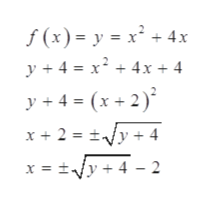# For the quadratic function f(x)=x^2+4x, answer part (a) below​(a) Determine the domain and the range of the function (write the answer in interval notation).

Question
3 views

​(a) Determine the domain and the range of the function (write the answer in interval notation).
check_circle

Step 1

Consider the given function.

Step 2

Part (a)

To find the domain of the given function.

Since the function f(x) is defined for all real values of x.

Therefore, the domain is all real values.

Step 3

Now, to find the range of the given function.

The values of the f...help_outlineImage Transcriptionclosef (x)= y x 4x +4 x24x+ 4 y4= y+4=(x+2) x2 = +4 y4 2 x = = fullscreen

### Want to see the full answer?

See Solution

#### Want to see this answer and more?

Solutions are written by subject experts who are available 24/7. Questions are typically answered within 1 hour.*

See Solution
*Response times may vary by subject and question.
Tagged in

### Other